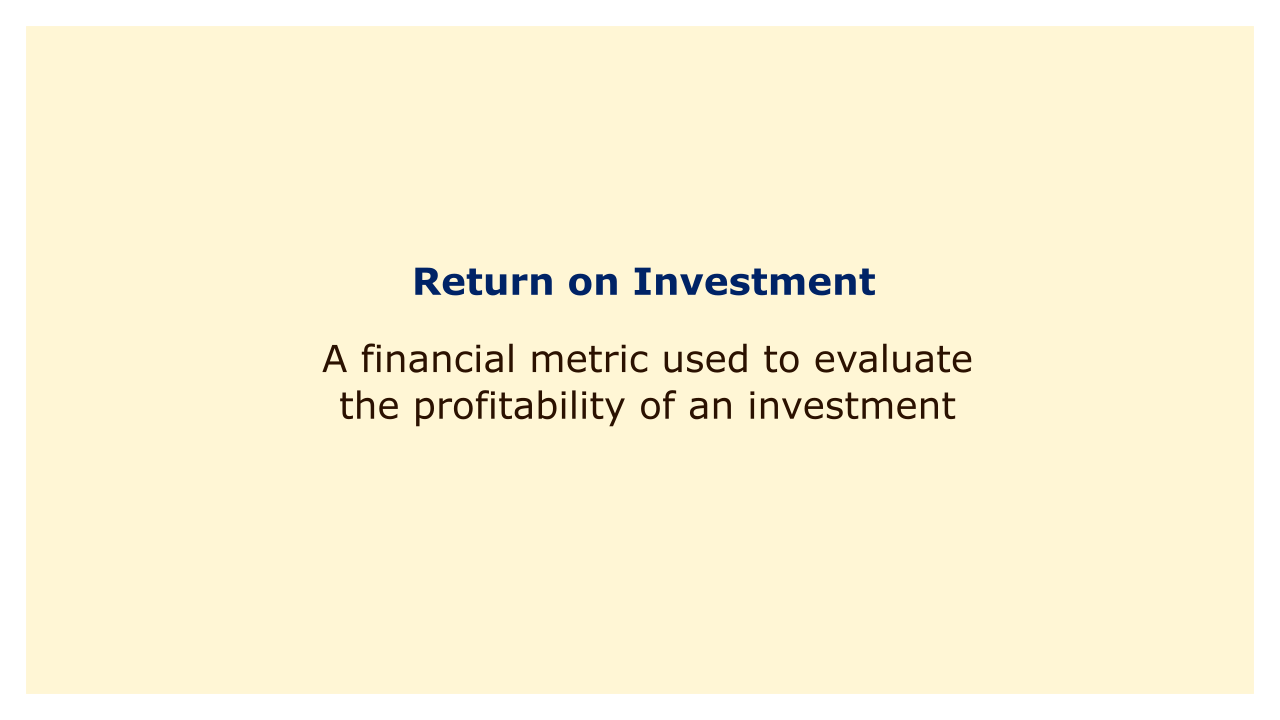# Return on InvestmentImage: Moneybestpal.com

### A financial metric called return on investment (ROI) is used to assess an investment's profitability. ROI gauges how much money is made back in relation to the cost of the investment. ROI can be stated as a ratio or a percentage.

The formula for ROI is:

ROI = (Net Profit / Cost of Investment) x 100%

where Net Profit is the total return on the investment minus the cost of the investment.

Due to its ease of use and capacity to evaluate the profitability of various assets, ROI is a frequently used financial indicator for investment evaluation. It does, however, have some restrictions. It does not account for factors like inflation, the time value of money, or the risk involved with an investment, for instance. In order to provide a more thorough study of an investment, it should be utilized in conjunction with other financial measures.
Tags